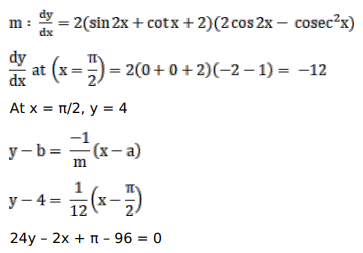# Find the equation of the normal to the curveQuestion:

Find the equation of the normal to the curve $y=(\sin 2 x+\cot x+2)^{2}$ at $x=\frac{\pi}{2}$

Solution: